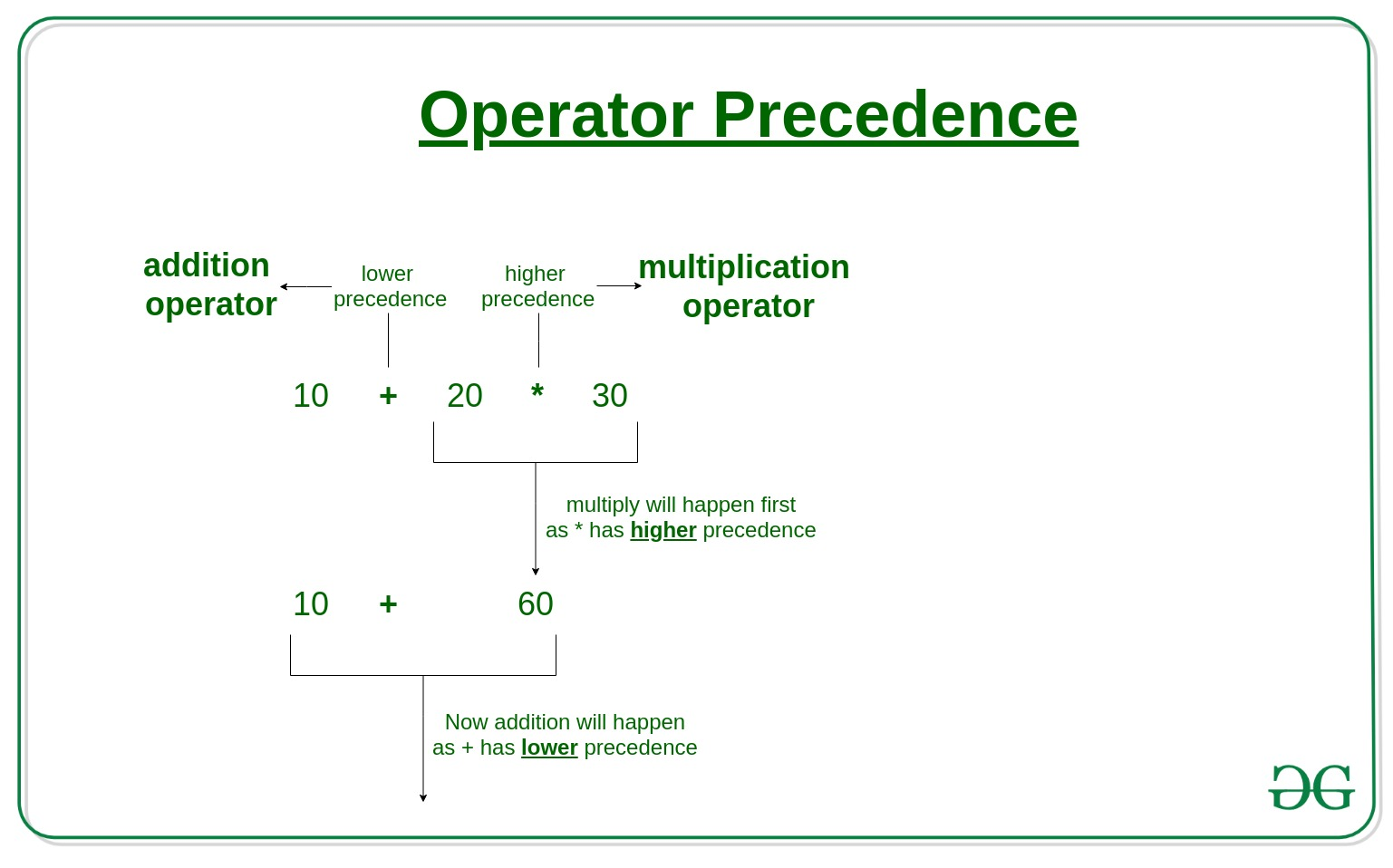GFG App
Open AppBrowser
Continue

# Operator Precedence in Ruby

Operators are used to perform different kinds of operations on operands. Which operator is performed first in an expression with more than one operators with different precedence is determined by operator precedence. when two operators of the same precedence appear in expression associativity is used. For example, ‘*’ and ‘/’ have the same precedence, so the expression “100 / 10 * 10” is worked as “(100 / 10) * 10”.```10 + 20 * 30 is calculated as 10 + (20 * 30)
and not as (10 + 20) * 30```

In below table operators with the highest precedence appear at the top of the table and operators with the lowest precedence appear at the bottom.

Operator

Category
[ ] [ ]= Element reference, element set
** Exponentiation
!, ~, + Boolean NOT, bitwise complement, unary plus
*, /, % Multiplication, division, modulo (remainder)
+, – Addition (or concatenation), subtraction
< <, > > Bitwise shift-left (or append), bitwise shift-right
& Bitwise AND
|, ^ Bitwise OR, bitwise XOR
>, >=, <, <= Ordering
<=>, ==, ===, !=, =~, !~ Equality, pattern matching, comparison
&& Logical AND
|| Boolean OR
| | Logical OR
.., …= Range creation and Boolean flip-flops
?, : Conditional
modifier-rescue Exception-handling modifier
=, +=, -=, etc. Assignment
defined? Test variable definition and type
not Boolean NOT (low precedence)
or, and Boolean OR, Boolean AND
modifier-if, modifier-unless, modifier-while, modifier-until Conditional and loop modifiers
begin/end blocks

Below is the example of operator Precedence.
Example :

 `# Ruby program to show Operators Precedence  ` ` `  `     ``a = ``20``;  ` `     ``b = ``10``;  ` `     ``c = ``15``;  ` `     ``d = ``5``;  ` `     ``e = ``0` `         `  `    ``# operators with the highest precedence  ` `    ``# will operate first  ` `    ``e = a + b * c / d;  ` `             `  `        ``# step 1: 20 + (10 * 15) /5  ` `        ``# step 2: 20 + (150 /5)  ` `        ``# step 3:(20 + 30) ` `             `  `    ``puts``"Value of a + b * c / d is : #{e}"`

Output :

`Value of a + b * c / d is : 50`

In above example, multiply and divide have equal precedence. so it will work like 20 + (10 * 15) /5. firstly multiply perform than divide after that addition because addition has low precedence.

My Personal Notes arrow_drop_up